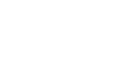Railo 4.2 Reference
Choose a function:

## Function IMAGEDRAWCUBICCURVE

Draws a cubic curve.

## Example

`imagedrawcubiccurve(any name,number x1,number y1,number ctrlx1,number ctrly1,number ctrlx2,number ctrly2,number x2,number y2):void`

## Arguments

The arguments for this function are set. You can not use other arguments except the following ones.
Name Type Required Description
name any  Yes The image on which this operation is performed.
x1 number  Yes The x coordinate of the start point of the cubic curve segment.
y1 number  Yes The y coordinate of the start point of the cubic curve segment.
ctrlx1 number  Yes The x coordinate of the first control point of the cubic curve segment.
ctrly1 number  Yes The y coordinate of the first control point of the cubic curve segment.
ctrlx2 number  Yes The x coordinate of the second control point of the cubic curve segment.
ctrly2 number  Yes The y coordinate of the second control point of the cubic curve segment.
x2 number  Yes The x coordinate of the end point of the cubic curve segment.
y2 number  Yes The y coordinate of the end point of the cubic curve segment.